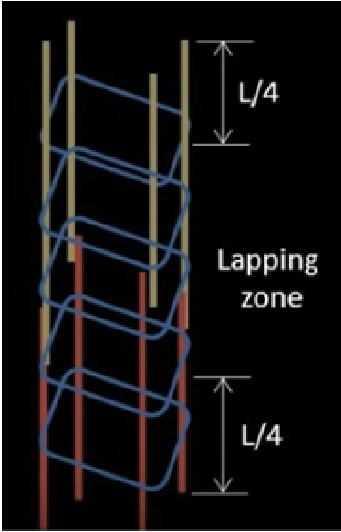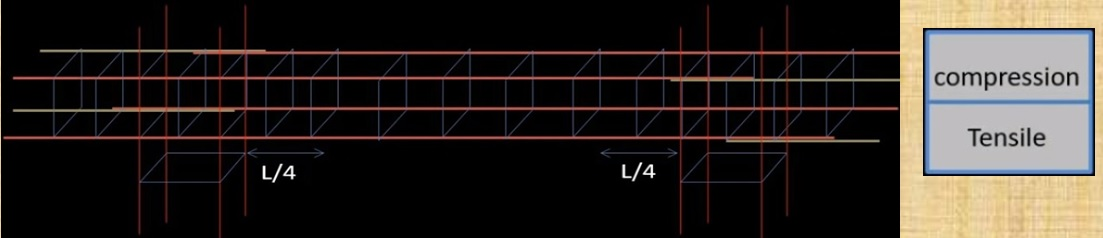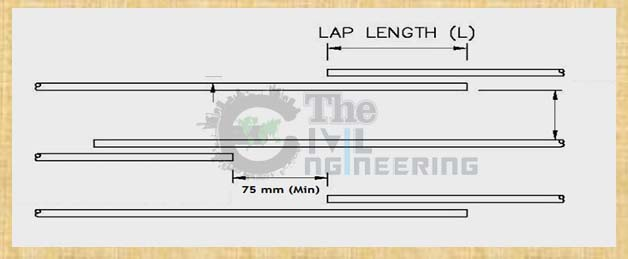# How to Calculate Lap Length in Column Beam and Slab | Lapping Zone of Column Beam Slab and Footing:

In this Article today we will talk about the How to Calculate Lap Length in Column Beam & SlabLapping Zone of ColumnLapping Zone of BeamLapping Zone of Slab | Lap Length FormulaWhat is Lap Length | Common Lap Length as per IS 456 | Why we Provide Lap Length | Difference between Lap Length and Development Length | Reinforcement Lap Length | Lap Length for Footing

## What is Lap Length?

Lap Length is required when bars placed short of their required length (due to nonavailability of longer bars) need to be extended.

• Lap Length is also required when the bar diameter has to be changed along the length (as is sometimes done in columns).Branding

The purpose of ‘Lap’ is to transfer the axial force effectively from the terminating bar into the connecting bar with this same line of action in the junction. This invariably introduces stress concentrations at this surrounding concrete. These effects should be minimized by

• Using proper splicing techniques.
• Keeping these lapping locations away from sections with high flexural/shear stresses. and
• Staggering the locations of splicing at the individual bars of a group (as typically in a column).

When splicing in such situations becomes unavoidable, special precautions need to be employed, such as

• Increasing the length of the lap (In lap splices and lap welding)
• Using spirals or closely-spaced stirrups around the length of the stirrups.

### Overview:

It is a general practice to manufacture the reinforcement steel bars in 12m length so as to ensure ease in transportation and handling.

However, during the construction of reinforced concrete structures; larger beams, columns, and slabs may be required.

In such a case, the reinforcement bars have to be overlapped to get the desired length.

Usually, such overlapping of bars is done where the magnitude of the bending stress is least.

When the two reinforcement bars have an equal diameter; the lap length can be calculated using the following formula:

Lap Length = 50 x D

Where, D = diameter of the reinforcement bars

In case, the diameters of the reinforcement bars are not equal then the lap length is calculated using the value of the bar with a smaller diameter. Calculate Lap Length in Column Beam & Slab

#### a. Lap Length in Tension:

For the lap length in tension, the following formula can be used for calculating the lap length including the anchorage value of the hooks,

1. For flexural tension, Lap length = L x d or 30 x d (The greater value among the two values calculated is taken.)

2. For direct tension, Lap length = 2 x L x d or 30 x d (The greater value among the two values calculated is taken.)

Where,

L = development length

In such a case, the straight lapping length of the reinforcement bars must be greater than 200mm or 15 x d. Calculate Lap Length in Column Beam & Slab formula

#### b. Lap Length in Compression

For the lap length in compression, the value of the lap length can be taken the same as that of the development length.

However, in no case, the lap length can be less than 24 x d.

## Why Do We Provide Lap Length ???

Reinforcement steel is generally manufactured for a standard 12m length. During construction when steel is to be provided for a length greater than 12m, the bars are lapped to maintain continuity in the reinforced structures. This lap length varies in different RCC structures and generally lies between 30d (d=bar diameter) to 55d depending on the design and grade. Calculate Lap Length in Column Beam & Slab

### Importance of Providing Lap Length

The lap length is necessary for reinforced concrete structures to allow the transfer of both tensile and compressive loads from one reinforcement bar to another by means of shear or skin friction.

The absence of lap length may disrupt the load transfer mechanism and lead to the failure of the entire structure. Lapping Zone of Column beam slab footing

Also, when the lap length provided is not adequate, the reinforcement bars may split thereby leading to the formation of cracks in concrete.

Thus, the lap length is necessary for reinforced concrete structures.

## What will happen if we don’t Provide Lap Length?

As we know that lapping should be provided between two rebar in order to safely transfer shear forcess from one bar to next bar, if we don’t provide lap length between two rebar the load is not safely transferred to one bar to next bar, causing structure will be failed, if not failed it cause cracking, and permanent collapsing of a structure. For preventing from any cracking, structure failure and permanent collapsing, lapping should be provided in steel when we placed Steel in RCC structure. Common Lap Length as per IS 456

## Lap Length As Per IS 456:

Generally, development length is 41d where d is the diameter of the bar. For direct tension, the lap length should be 2 Ld or 30d whichever is greater is considered. In this case, the straight length of the lapping bar shall not be less than 15d or 20cm. Common Lap Length as per IS 456

## Lap Length of Columns:

The codal provision for calculating the lap length of columns in a reinforced concrete structure is given in CL. 26.5.3 of IS 456:200.

According to this code, the diameter of the bars must not be less than 12mm.

The number of longitudinal bars that must be provided in a rectangular column must be equal to or greater than four and equal to or greater than 6 in a circular column.

The spacing of such longitudinal bars should be lesser than 300mm when measured along the periphery of the column.

The lap length of columns can be calculated using the following formula,

Lap Length of Column = 45 x d

where; Common Lap Length as per IS 456

d = diameter of the bar Common Lap Length as per IS 456

### Important Point Should be Remember for Column Lap Length:• Lapping should be provided at the center of column because bending moment at mid point is zero so try to lap at mid point. Common Lap Length as per IS 456
• Lapping of bars should be provided alternately. Lap should not be given at same point because buckling may occur.
• Lateral ties should be provided closely spaced in Lapping zone.
• Due to maximum stress we can’t lap at column/beam/slab joint. Lapping should not be given at L/4 distance from top and bottom of the slab. Why we Provide Lap Length

## Lap Length of Beams:

The codal provision for calculating the lap length of beams in reinforced concrete structure is given in CL. 26.5.2 of IS 456:200.

According to this code, side reinforcement bars must be provided in case the depth of the web of the beam is greater than 75 cm.

In such a case, the area of the reinforcement bars used must be greater than 0.1 percent of the total area of the web.

The reinforcement bars must be equally distributed on both faces of the beam such that the spacing is not greater than 300 mm or the thickness of the web, whichever has a lesser value.

In beams, the transverse reinforcements must be provided such that they lie around the exterior tension and compression bars.

In T-beams and I-beams, such reinforcement shall pass around longitudinal bars located close to the outer face of the flange. The lap length of beams can be calculated using the following formula, Why we Provide Lap Length

Lap Length of Beams = 60 x d

### Important Point Should be Remember for Beam Lap Length:• Lapping (24d) in top bars avoided L/3 distance from both end. For top bar lapping should be at mid span.
• Lapping (45d) in bottom bars lap should be provided at column junction or L/4 distance from column face but should not be in mid span of beam.
• Stirrups should be closely spaced near the columns and lose/normal at mid span.
• Lapping of bars should be alternately provided. Why we Provide Lap Length

## Lap Length of Slabs:

The codal provision for calculating the lap length of slabs in reinforced concrete structures is given in CL. 26.5.1 of IS 456:200.

According to this code, the diameter of the reinforcement bars must be lesser than one-eighth of the total slab thickness.

The lap length of slabs can be calculated using the following formula,

Lap Length of Slab = 60 x d Difference between Lap Length and Development Length

### Important Point Should be Remember for Slab Lap Length:• RCC slabs function is similar to beams if the slab is designed as one way. It is ideal to lap bars at point of least bending moment or at points of contra flexure.
• Practically laps are provided beyond L/5 to L/3 ( L being the effective span) from a support for bars at bottom of slab. The top bars are generally short and no laps are necessary. However at no point the lapped bars shall exceed one third of the total bars.
• Bars will be considered staggered if end to end distance b/w laps is at least (lap length +75mm) Difference between Lap Length and Development Length

## Lap Length for Footing:

If it’s a dowel reinforcement then lap length for footing shall be 12D and If reinforcement is subjected to tension (within the tension chord) then lapping length shall be 50D. Actual lap length depends on diameter, coating, steel grade, cover, concrete grade and actual steel stress that needs to be transferred. Reinforcement Lap Length

## What is Minimum Lap Length:

Minimum lap length for direct tension is equal to development length,it should be not less than 15d or 20cm. And for compression, minimum lab length should be taken as not less than 24d. Where d is diameter of rebar Reinforcement Lap Length

## Lapping Zone:

### Lapping Zone for Column:

This is the column. L is the length of the column. In the case of the column, the tension zone is located at an L/4 distance from both ends of the column. This zone experiences tension so here we should not provide lapping.

The bending moment at the middle portion of the column is zero it means the middle portion of the column is least stressed. Hence, lapping should be provided in the mid-section of the column so that transfer of stresses from bar to bar happens smoothly in this region. Lap Length for Footing

### Lapping Zone for Beam:

Whereas in the case of the beam as I already explained before the top portion of the beam experiences compression and the bottom portion experiences tension. So, the top reinforcement in the beam is left at the midspan. As the beam does not experience any negative moment at midspan so lapping is great in this region.

In the case of bottom reinforcement, the lapping is provided near the ends of the beam or L/4  distance from column face but should not be in the midpoint of the beam, and one last point The lapping should not be provided at joints. Lap Length for Footing

### Lapping Zone for Slab:

The function of the RCC Slab is similar to the beam If it is designed in One Way Slab. The perfect position for lapping is at where the bending moment is least, the point of contra flexure. For bottom reinforcement, laps are provided L/5 to L/3 distance from the support. ( L = eective span) For top reinforcement, no lap is required since they are generally short. Although In no case, the lapped bars should not exceed one-third of the total bars.

In the two-way slab, the same practice as mentioned above should be followed for both directions.

If an end-to-end distance between the lap is the least, then the lap should be provided in a staggered manner. ( lap length +75 mm)

Generally, the lap length provided for the slab 60d, column 45d, and the beam is 60d for the M20 grade of concrete. Lap Length for Footing

## Difference Between Lap Length and Development Length:

 S.N. Lap Length Development Length 1 It refers to the overlapping length of two reinforcement bars for achieving the desired design length. It refers to the length that is necessary for transferring the stress imposed on the concrete member. 2 Lapping is done in reinforced concrete structures, to achieve the required design length of the reinforcement bars. Development length is necessary to ensure that the necessary bond strength between the concrete and the embedded reinforcement bar is achieved.

## FAQs:

Q. Determine the lap length for two bars with a diameter of 40mm.

Solution,

When the two bars have the same diameter, the lap length can be calculated as,

Lap Length = 50 x d = 50 x 40 = 2000 mm = 2 m

Q. Determine the lap length for two bars in which the diameter of one bar is 25mm and another of diameter 40mm.

Solution,

When two bars have a different diameter, the smaller diameter must be used i.e.

Lap Length = 50 x d = 50 x 25 = 1250 mm = 12.5 m

Q. Determine the lap length for beam, slab (Take the diameter of bar = 12 mm) and the lap length for column (Take the diameter of bar = 24 mm).

Solution,

i. Lap Length of beam = 60 x d = 60 x 12 mm = 720 mm

ii. Lap Length of slab = 60 x d = 60 x 12 mm =720 mm

iii. Lap Length of column = 45 x d = 45 x 24 mm = 1080 mm

Q. Determine the lap length for the following:

i. Nominal Mix of 1:2:4, if the diameter of the bar is 20 mm.

Lap Length for 1:2:4 concrete mix = 40 x D = 40 x 20 mm = 800 mm

ii. Nominal Mix of 1:1.5:3 for the column, if the diameter of the bar is 20 mm.

Lap Length for 1:1.5:3 concrete mix for column = 45 x D = 45 x 20 mm= 900 mm

iii. Nominal Mix of 1:1.5:3 for beam, if the diameter of the bar is 20 mm.

Lap Length for 1:1.5:3 concrete mix for beam = 60 x D = 60 x 20 mm = 1200 mm

iv. Nominal Mix of 1:1.5:3 for the slab, if the diameter of the bar is 20 mm.

Lap Length for 1:1.5:3 concrete mix for slab = 60 x D = 60 x 20 mm = 1200 mm

## Conclusion:

Full article on Lap Length in Column Beam & Slab | Lapping Zone of Column | Lapping Zone of Beam | Lapping Zone of Slab | Lap Length Formula | What is Lap Length | Common Lap Length as per IS 456 | Why we Provide Lap Length | Difference between Lap Length and Development Length | Reinforcement Lap Length | Lap Length for Footing. Thank you for the full reading of this article in “The Civil Engineering” platform in English. If you find this post helpful, then help others by sharing it on social media. If any formula of BBS is missing from this article please tell me in comments.

1.Musembi Malinda
2.ELMER ANDRIAMIZAKA